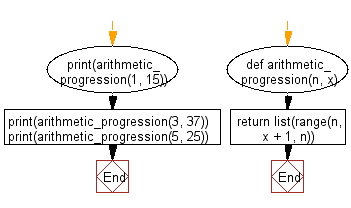﻿ Python: Generates a list of numbers in the arithmetic progression within a range - w3resource# Python: Generates a list of numbers in the arithmetic progression within a range

## Python List: Exercise - 272 with Solution

An Arithmetic progression (AP) or arithmetic sequence is a sequence of numbers such that the difference between the consecutive terms is constant. For instance, the sequence 5, 7, 9, 11, 13, 15, . . . is an arithmetic progression with a common difference of 2.
If the initial term of an arithmetic progression is a1 and the common difference of successive members is d, then the nth term of the sequence (an) is given by:

an = a1 + (n - 1)d,

and in general

an = am + (n - m)d.

A finite portion of an arithmetic progression is called a finite arithmetic progression and sometimes just called an arithmetic progression. The sum of a finite arithmetic progression is called an arithmetic series.

Write a Python program to generate a list of numbers in the arithmetic progression starting with the given positive integer and up to the specified limit.

Use range() and list() with the appropriate start, step and end values.

Sample Solution:

Python Code:

``````def arithmetic_progression(n, x):
return list(range(n, x + 1, n))

print(arithmetic_progression(1, 15))
print(arithmetic_progression(3, 37))
print(arithmetic_progression(5, 25))
```
```

Sample Output:

```[1, 2, 3, 4, 5, 6, 7, 8, 9, 10, 11, 12, 13, 14, 15]
[3, 6, 9, 12, 15, 18, 21, 24, 27, 30, 33, 36]
[5, 10, 15, 20, 25]
```

Flowchart:## Visualize Python code execution:

The following tool visualize what the computer is doing step-by-step as it executes the said program:

Python Code Editor:

Have another way to solve this solution? Contribute your code (and comments) through Disqus.

What is the difficulty level of this exercise?

Test your Python skills with w3resource's quiz

﻿

## Python: Tips of the Day

```print(2_000_000)
```2000000